## Example Questions

1 3 Next →

### Example Question #1631 : Psat Mathematics

Out of 85 students in a certain class, 42 own a laptop and 54 own an mp3 player. If 5 students don't own either, what fraction of the students own both a laptop and an mp3 player?

16/85

7/40

1/10

1/8

19/80

16/85

Explanation:

Once you subtract the 5 students that don't own either, there are 80 students left.

There's 96 total students when you add the number that own an mp3 and the number that own a laptop, meaning 16 own both.

Recall that the fraction will be number of students who have both laptop and mp3 divided by the total students in the class.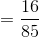### Example Question #88 : Proportion / Ratio / Rate

For every 5 glasses of lemonade, Bud earns $2. How many glasses must he sell to earn$24?Explanation:

We can set up a simple proportion to solve this. For every 5 glasses, Bud gets $2. For every x glasses, he earns$24.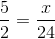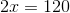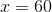### Example Question #89 : Proportion / Ratio / Rate

Mindi can take down seven museum displays every half hour. How many displays can she take down completely in an hour and fifteen minutes?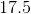Explanation:

Let's set up a proportion to solve this problem, like so: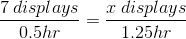We can cross-multiply and solve for the unknown. We can ignore our units for now as the answer's units will result in displays as the unit.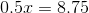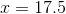This means that Mindi can take downdisplays in the given time. However, the question asks for the number of displays she can take down completely in the given time. This means that our answer is rounded down to### Example Question #90 : Proportion / Ratio / Rate

A rabbit runs at a speed ofmiles per hour. How long does it take for it to runmiles?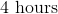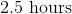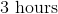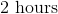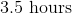Explanation:

Let's set up a proportion to help solve this problem. We can say: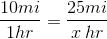Now, we can cross-multiply and solve for the unknown. Don't worry about units for now. They will cancel out and leave us with hours as the units for our answer.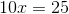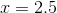Therefore, our answer ishours.

1 3 Next →